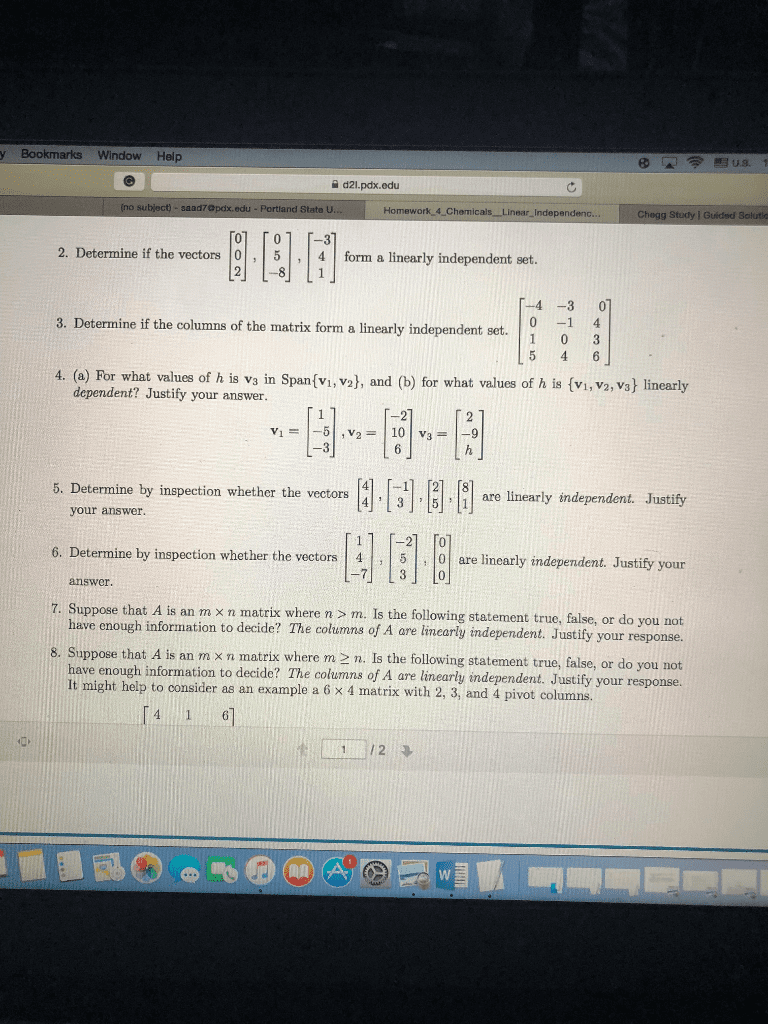1
0
watching
119
views10 Nov 2019

# questions 2,4,6,8y Bookmarks Window Help d21.pdx.edu saad7Opdx.edu 1 o 2. Determine if the vectors 5 4 form a linearly independent set. 2 81 4-3 0 3. Determine if the columns of the matrix form a linearly indepenset.03 -1 4 ? / 4. (a) For what values of h is va in Span(vi, v2), and (b) for what values of h is (vi, v2, vs linearly dependent? Justify your answer. -3 5. Determine by inspection whether the vectors3 4] -1 [21 8 are linearly independent. Justify iearly mdependent Justify your answer. 20 73 o 7. Suppose that A is an m x n matrix where n 3 m. Is the followi 6. Determine by inspection whether the vectors 4 5 o are linearly indepe answer ng statement true, false, or do you not have enough information to decide? The columns of A are linearly independent. Justify your response. 8. S appose that A is an m x n matrix where m 2 n. Is the following statement true, false, or do you not have enough information to decide? The columns of A are linearly independent. Justify your response. It might help to consider as an example a 6 x 4 matrix with 2, 3, and 4 pivot columns. 112